### IMO Shortlist 2008 problem G3

Kvaliteta:
Avg: 3,0
Težina:
Avg: 7,0
Let$ABCD$ be a convex quadrilateral and let$P$ and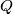$Q$ be points in$ABCD$ such that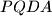$PQDA$ and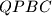$QPBC$ are cyclic quadrilaterals. Suppose that there exists a point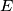$E$ on the line segment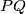$PQ$ such that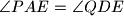$\angle PAE = \angle QDE$ and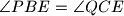$\angle PBE = \angle QCE$. Show that the quadrilateral$ABCD$ is cyclic.

Proposed by John Cuya, Peru
Izvor: Međunarodna matematička olimpijada, shortlist 2008